Homework Explained - Math Practice 101Dear guest, you are not a registered member. As a guest, you only have read-only access to our books, tests and other practice materials.

As a registered member you can:

Registration is free and doesn't require any type of payment information. Click here to Register.
Go to page:
Chapter 7: Geometric Figures; Lesson 1: Classify Angles

### Independent Practice

Name each angle in four ways. Then classify the angle as acute, right, obtuse, or straight

• Question 1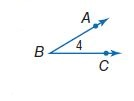• Question 2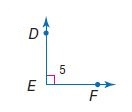• Question 3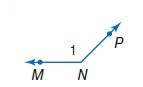Identify Structure Refer to the diagram at the right. Identify each angle pair as adjacent, vertical, or neither.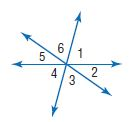• Question 4

$$\angle 2$$ and $$\angle 5$$

• Question 5

$$\angle 4$$ and $$\angle 6$$

• Question 6

$$\angle 3$$ and $$\angle 4$$

• Question 7

$$\angle 5$$ and $$\angle 6$$

• Question 8

$$\angle 1$$ and $$\angle 3$$

• Question 9

$$\angle 1$$ and $$\angle 4$$

• Question 10

What is the value of x in the figure at the right?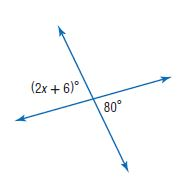•  $$x=$$
• Question 11

What is the value of x in the figure at the right?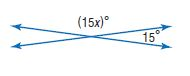•  $$x=$$

Yes, email page to my online tutor. (if you didn't add a tutor yet, you can add one here)